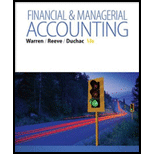# Five measures of solvency or profitability The balance sheet for Garcon Inc. at the end of the current fiscal year indicated the following: Bonds payable, 8% $5,000,000 Preferred$4 stock, $50 par 2,500,000 Common stock,$ 10 par 5,000,000 Income before income tax was $3,000,000, and income taxes were 51,200,000 for the current year. Cash dividends paid on common stock during the current year totaled$1,200,000. The common stock was selling for $32 per share at the end of the year. Determine each of the following: (A) times interest earned ratio (B) earnings per share on common stock, (C) price-earnings ratio, (D) dividends per share of common stock, and (E) dividend yield. (Round ratios and percentages to one decimal place, except for per-share amounts.)BuyFindarrow_forward ### Financial & Managerial Accounting 13th Edition Carl Warren + 2 others Publisher: Cengage Learning ISBN: 9781285866307 #### Solutions Chapter SectionBuyFindarrow_forward ### Financial & Managerial Accounting 13th Edition Carl Warren + 2 others Publisher: Cengage Learning ISBN: 9781285866307 Chapter 15, Problem 15.20EX Textbook Problem 1 views ## Five measures of solvency or profitabilityThe balance sheet for Garcon Inc. at the end of the current fiscal year indicated the following: Bonds payable, 8%$5,000,000 Preferred $4 stock,$50 par 2,500,000 Common stock, $10 par 5,000,000 Income before income tax was$3,000,000, and income taxes were 51,200,000 for the current year. Cash dividends paid on common stock during the current year totaled $1,200,000. The common stock was selling for$32 per share at the end of the year. Determine each of the following: (A) times interest earned ratio (B) earnings per share on common stock, (C) price-earnings ratio, (D) dividends per share of common stock, and (E) dividend yield. (Round ratios and percentages to one decimal place, except for per-share amounts.)

To determine

Financial Ratios: Financial ratios are the metrics used to evaluate the liquidity, capabilities, profitability, and overall performance of a company.

Given info: Income before income tax and interest expense.

The following ratios:

1. a.      Times interest earned ratio.
2. b.      Earnings per share on the common stock.
3. c.       Price earnings ratio.
4. d.      Dividend per share of common stock.
5. e.       Dividend yield.

### Explanation of Solution

a.

Times interest earned ratio quantifies the number of times the earnings before interest and taxes can pay the interest expense. First, determine the sum of income before income tax and interest expense. Then, divide the sum by interest expense.

Formula:

Times-interest-earned ratio }=Income before income tax+Interest expenseInterest expense

Times interest earned ratio:

Times-interest-earned ratio }=Income before income tax+Interest expenseInterest expense=$3,000,000+$400,000$400,000=8.5times Working note for determining the interest expense: Interest expense = Bonds payable × Interest rate=$5,000,000 × 8%= $400,000 b. Earnings per share help to measure the profitability of a company. Earnings per share are the amount of profit that is allocated to each share of outstanding stock. Formula: Earningspershare = Netincome – PreferreddividendsWeighted average number of common shares outstanding Earnings per share on the common stock: Earnings per share=[Net incomePreferred dividends(Weighted average shares of common stock outstanding)]=$1,800,000$20,000500,000shares=$1,600,00050,000shares=$3.20 Working note for determination of preferred dividends and weighted average number of common shares outstanding are provided below: Preferred dividends = Preferred stockParvalue × Stock value$2,500,000$50×$4=$200,000 Weighted average number of common shares }Total value of shares Par value per share=$5,000,000\$10=500,000shares

c

### Still sussing out bartleby?

Check out a sample textbook solution.

See a sample solution

#### The Solution to Your Study Problems

Bartleby provides explanations to thousands of textbook problems written by our experts, many with advanced degrees!

Get Started

Find more solutions based on key concepts
Conciseness es valued in business. However, can messages be too short?

Essentials of Business Communication (MindTap Course List)

What is OSI?

Accounting Information Systems

ROE AND ROIC Hilyard Industries net income is 25,000, its interest expense is 5,000, and its tax rate is 40%. I...

Fundamentals of Financial Management, Concise Edition (with Thomson ONE - Business School Edition, 1 term (6 months) Printed Access Card) (MindTap Course List)